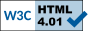Abstract Measures of
Low-Level Computational Complexity

Proposal

## The Project Proposal

In this project, the overall aim is to obtain a deep and thorough understanding of the abstract complexity inherent in bounded arithmetic theories and propositional proof systems. More specifically, this research will achieve this aim by:

#### Objective 1: Computational content of dynamic ordinals.

We wish to understand to what extent dynamic ordinals can be viewed as abstract measures of computational strength. This should link intrinsically definable functions of bounded arithmetic theories to the computation of dynamic ordinals via translation to propositional proof systems, cut-elimination and lower bounds. So far, dynamic ordinals have proven useful as abstract measures of proof complexity of bounded arithmetic theories.

#### Objective 2: Improvement of separation results.

Dynamic ordinal analysis builds on strong lower bounds for propositional proof systems. We want to improve existing lower bounds for a class of intensively studied propositional proof systems which are called constant depth LK proof systems. Our aim is to find separating principles of lower logical complexity than previously known ones. In particular, we want to settle the proof complexity of the Ramsey Principle.

#### Objective 3: Abstract measures of low-level computational complexity.

We want to achieve new abstract measures for bounded arithmetic theories on the basis of previously developed new lower bounds, which characterise levels of provability other than dynamic ordinals, and which will characterise other levels of definable functions. This way, we hope to obtain a good characterisation of the ∑b1-definable functions of S32. We will derive new independence results for bounded arithmetic theories by using translations to propositional proof systems and new lower bounds, which are inherent in the new abstract measures.

 websites: Arnold Beckmann 2014-03-14